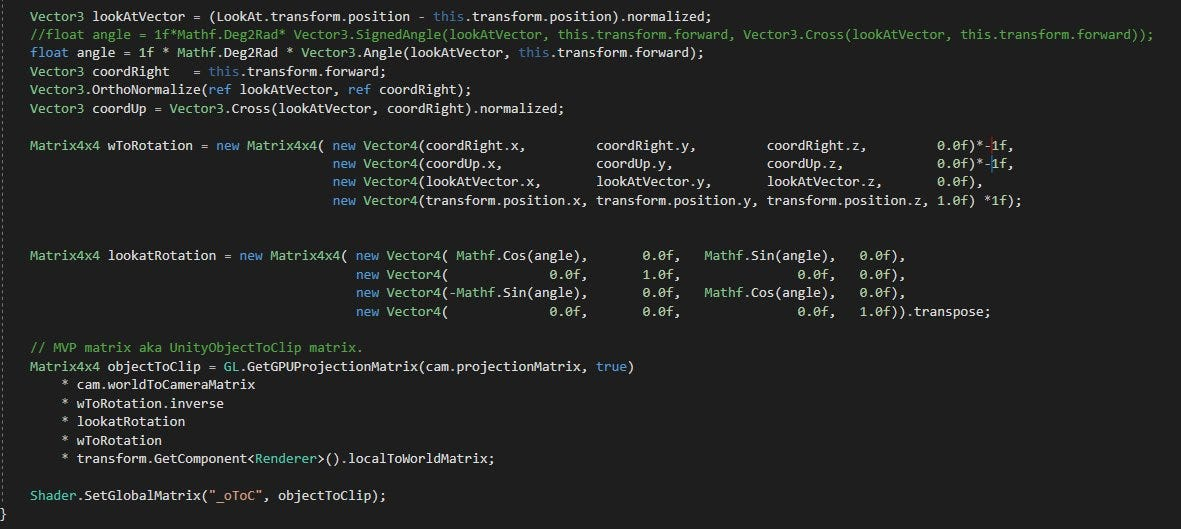# Motivation

The other day, I was thinking about making a horror like VR mini experience. In the exprience the statues look at you when you are not directly looking at them, and look away once you do. I got the inspiration from an art installation in Berlin. As this idea was added to the long and ever increasing list of 'To Do Projects’, I started thinking about how I would implement this.

# Gram Schmidt

My first idea was to use Gram Schmidt to create an orthonormal coordinate system, in which I can rotate the head in a way, that the forward vector of the head aligns with the LookAt vector. I won’t duel on this method, because I switched to using cross products. I will post the code though, so you can have a look at this as an alternative.Figure -3- Code for the Gram Schmidt process of constructing a rotation coordinate system

# Cross Products

As I was playing around with Gram Schmidt, I realized there is no reason to create a coordinate system to rotate the mesh, transform in that coordinate system, rotate and then transform back to world space. If I transform the mesh in a space, which has a column space where the z axis aligns with the LookAt vector, I can simply transform the coordinates from here to the clip space of the camera and everything will look fine. If this is not making any sense to you, I suggest reading my post on affine and non affine transformation matrices.

`Matrix4x4 objectToClip = GL.GetGPUProjectionMatrix(cam.projectionMatrix, true) * cam.worldToCameraMatrix * modelFoward.localToWorldMatrix * lookAtMatrix* modelFoward.worldToLocalMatrix * renderOfTheMesh.localToWorldMatrix;`

## Mesh Model Matrix

In the way Unity is set up (column major form), the matrix which is the most right is the transformation that happens first. This is the localToWorldMatrix of the renderer of our mesh. Do we need this transformation? I wanted to have this in, so that I can still rotate, scale and translate my mesh using the game object it is attached to. Specially for photogrammetry, since meshes tend to come in with the weirdest rotations based on the pipeline they went through. So that’s all this matrix does, it takes the transform of the mesh Game object and adds its transformation as the first thing to our matrix.

## ModelFoward WorldToLocalMatrix

How should our transformation know which way is forward for our statue? If we apply our Look At transfromation matrix simply in world space, it will make it so that the global forward, aligns itself with our LookAt vector. Since we want sForward to be aligned with LookAt, we first have to transform from world space to a space where sForward is the Z axis. This is what this world to local matrix does. I am simply using an empty game object for this. I rotate the empty game object in a way, so that its Z axis points toward what I think is foward for the statue (a vector that points where the statue is looking at). This vector also in codes the position of our pivot. It moves the mesh in a way, so that our pivot on the mesh, lay on the origin of our coordinate system. Since we wish to rotate around this pivot, after all your head also rotates around a point on your neck and not around your feet. If you want to know how to make this matrix yourself, look at my post on non affine matrices, I cover the construction of the model matrix there.

## LookAt Matrix

This is our actual look at. Making this matrix is super simple. You need to create a space, where the forward (Z-Axis) is the LookAt vector. The other two vector you should choose in a way, so that model up remains up. You can simply do this with three cross products.

`Vector3 lookAtVector = modelFoward.worldToLocalMatrix*(LookAt.transform.position — modelFoward.transform.position).normalized ; Vector3 coordRight = Vector3.Cross(Vector3.up, lookAtVector).normalized; Vector3 coordUp = Vector3.Cross(lookAtVector, coordRight).normalized;`

## ModelFoward LocalToWorldMatrix

Now that our look at is finished, we need to transform back in to world space from our pivot space. Why? Because we don’t know how to go directly from the pivot space in to camera space. Our camera is defined in terms of world coordinates. That is what this transformation does.

## VP Matrix

The last two matrices are the World to Camera and Projection matrix. This I have covered else where, all it does is brings our vertex coordinates from world space in the space which is used for displaying them. I am using the GL.GetProjectionMatrix, because unity documentation says I should (probably Camera.ProjectMatrix remains the same for different platforms or APIs, whereas the actual projection matrix which is used in the GPU is different for different scenarios).

# Vertex Shader and Blending

So we have our matrix, we pass it to the vertex shader. If we use this instead of UNITY_MATRIX_MVP, the entire mesh will be rotated around the pivot. This is not what we want, because only the head should rotate itself. I solved this by simply lerping between the two transformations based on the Y value of the vertex, but you can use vertex color or any other thing you can think of.

`       o.vertex   = mul(_oToC, float4(v.vertex.xyz, 1.)); float transition = smoothstep(-.2, 1., v.vertex.z);       o.vertex   = lerp( UnityObjectToClipPos(v.vertex), o.vertex, transition * _debugOrignalPosition);`

--

--